# Opamp Circuit confusion

• Engineering
Hi!
My task is to calculate gain A=Vo/Vin for circuit on scheme (OPAMPS are ideal):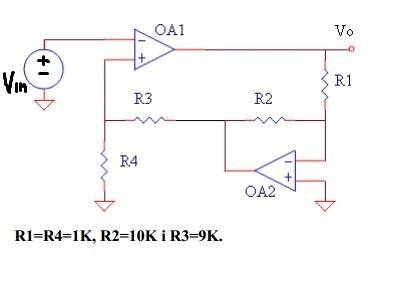Since OPAMPS are ideal, input currents are zero and V1=V2=Vin, V11=V22=0: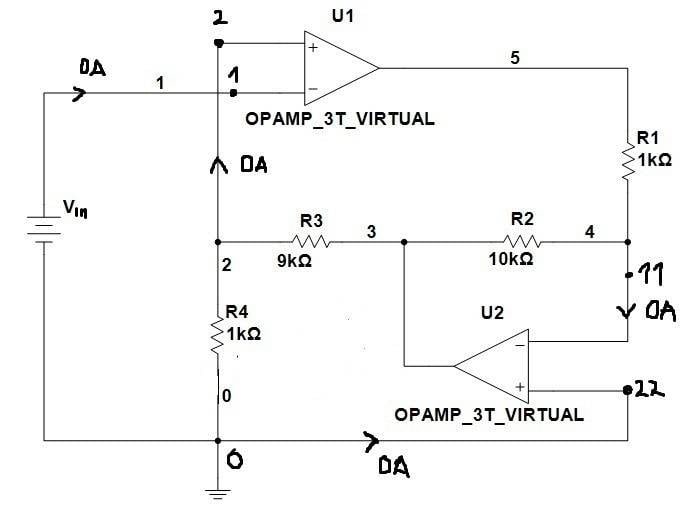KCL for node 0 suggests us that current through R4 (I4) must be zero: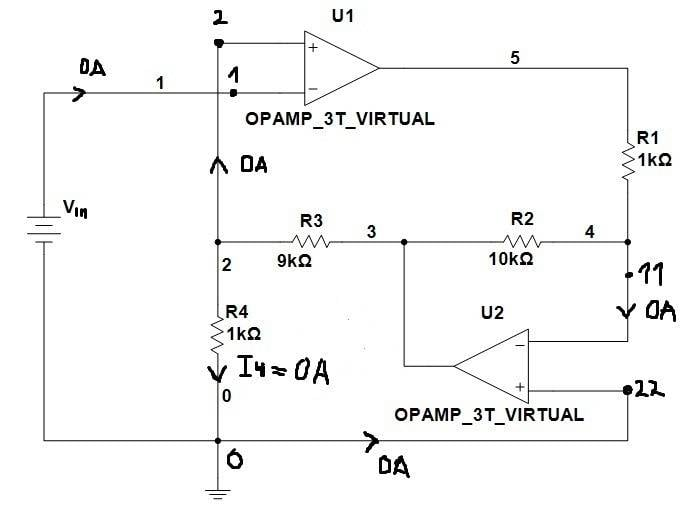On the other hand, we can calculate I4 as: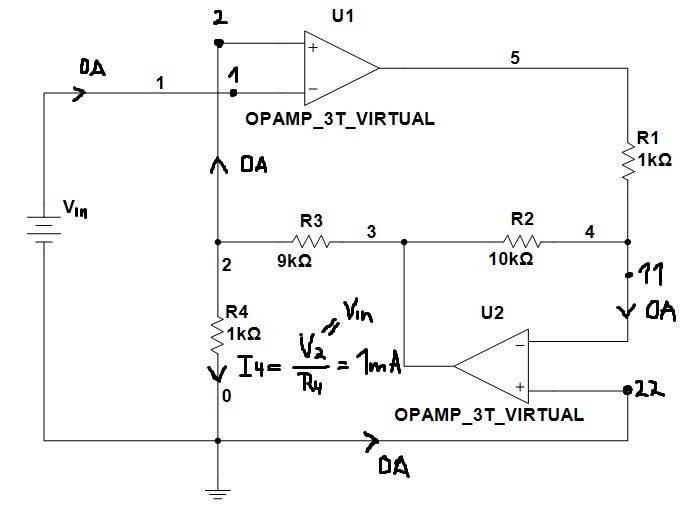I tested circuit using Multisim and I got this: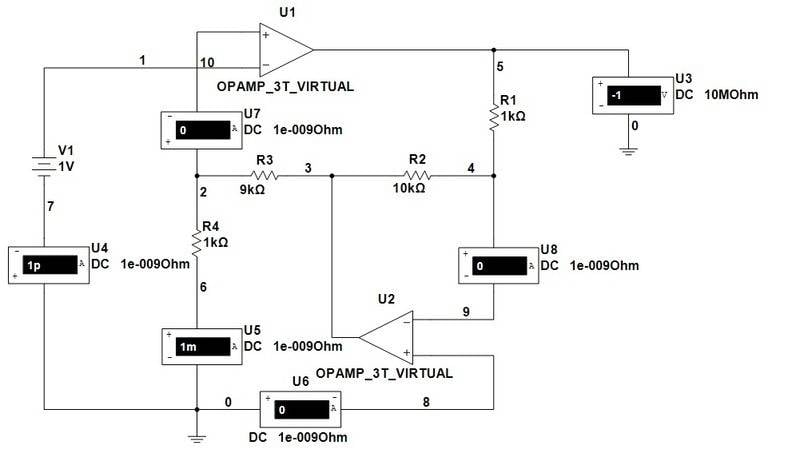For any input voltage Vin we have on output Vo=-Vin

berkeman
Mentor
Hi!
My task is to calculate gain A=Vo/Vin for circuit on scheme (OPAMPS are ideal):

KCL for node 0 suggests us that current through R4 (I4) must be zero:

View attachment 73245

I don't know where you get I4 = 0V in your 3rd figure. It's not zero, as your next calculation and the simulation show.

I would approach this problem by using voltage divider math. Label the central node voltage Va (the output of the right-hand opamp). Then write the KCL equation for that node. That gives you an equation that involves Va and Vin, and R2 and R3. Re-arrange that equation to give you Va in terms of Vin and those two resistors.

Then write the voltage divider equation for the left two resistors. Va is divided down to Vin by R3 and R4. Use those two equations to solve for Va in terms of Vin, and then use Va to calculate Vout.

Give that approach a try and see if it works for you...•1 person
berkeman
Mentor
BTW, it's best if you can make all of your opamp symbols have a consistent placement of the + and - inputs. The original figure used symbols with the - input on top and the + input on the bottom (which is the way I prefer to draw opamps). In your drawing tool, it looks like you rotated the standard opamp symbol instead of mirroring it, to make the right-pointing opamp. If possible, try to just use "mirror" instead of "rotate" when you make an opposite-facing symbol. Just a suggestion...I don't know where you get I4 = 0V in your 3rd figure.
Well, input resistances of ideal OPAMPs are infinite so input currents for both OPAMPs are zero and, since they are zero, I4 also must be zero (KCL equation for node 0: 0A+0A-I4=0, I4=0) ?

berkeman
Mentor
Well, input resistances of ideal OPAMPs are infinite so input currents for both OPAMPs are zero and, since they are zero, I4 also must be zero (KCL equation for node 0: 0A+0A-I4=0, I4=0) ?

I don't know where your "Node 0" is. But Va is not zero, and it has a path through resistors R3 and R4 to ground, so I4 will be non-zero.

Did you write the equations that I suggested? What solution did you find?

I marked node 0 with green colour: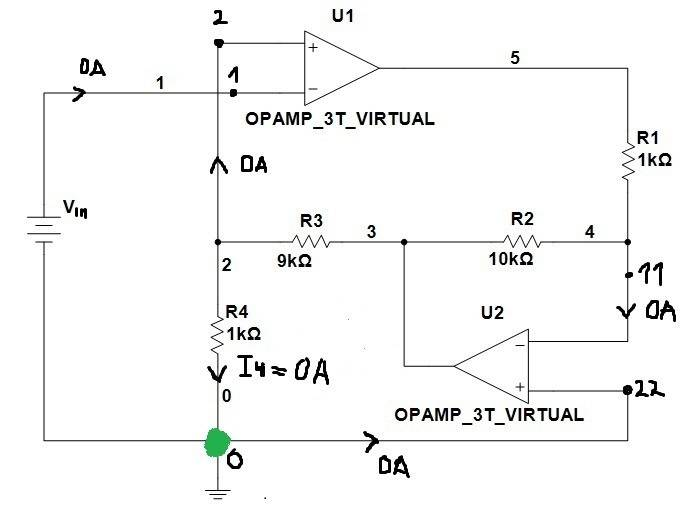I didn't try your method yet...

berkeman
Mentor
I marked node 0 with green colour:

View attachment 73268

I didn't try your method yet...

That point is ground. You don't usually write a KCL equation for the ground node when solving for a circuit. Let us know how my method works out for you.

I solved it using your method and I got correct result. Thanks :)
But I'm still confused about KCL for ground node. KCL should be always satisfied for every node.

berkeman
Mentor
I solved it using your method and I got correct result.
But I'm still confused about KCL for ground node. KCL should be always satisfied for every node. Thanks berkeman!

Great!As for the ground node, its KCL equation would be redundant with the other equations that you have written for the other nodes in the circuit. That's why folks don't bother to write a KCL eauation at the ground node -- it's just extra work that doesn't buy you anything.

donpacino
Gold Member
Hi!
My task is to calculate gain A=Vo/Vin for circuit on scheme (OPAMPS are ideal):

View attachment 73243

Since OPAMPS are ideal, input currents are zero and V1=V2=Vin, V11=V22=0: